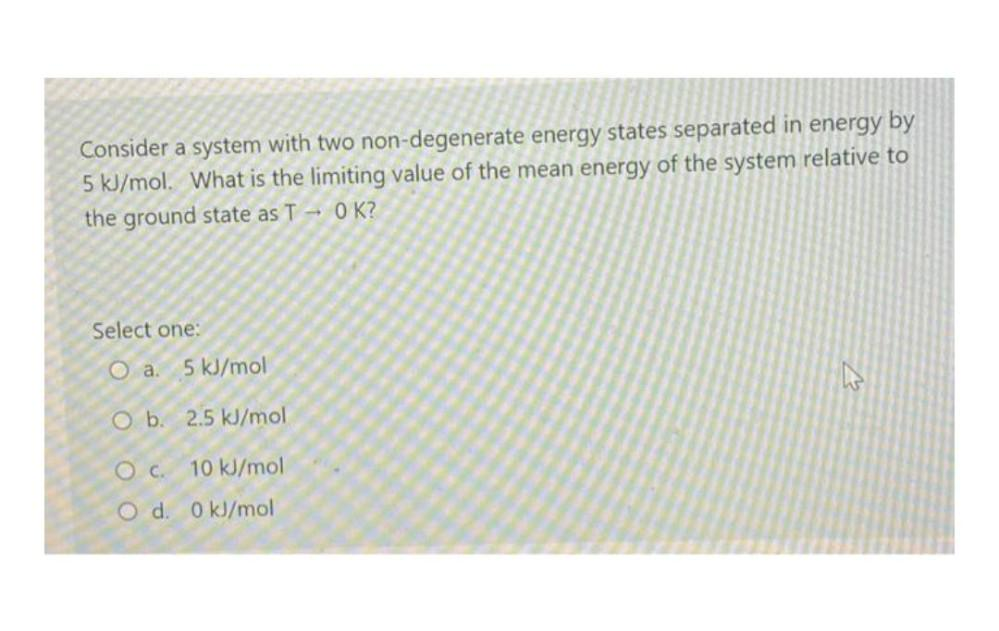Question:

# Consider a system with two non-degenerate energy states separated in energy by 5 kJ/mol. What is the limiting value of the meanConsider a system with two non-degenerate energy states separated in energy by 5 kJ/mol. What is the limiting value of the mean energy of the system relative to the ground state as T - OK? Select one: O a. 5 kJ/mol O b. 2.5 kJ/mol OC. 10 kJ/mol O d. 0 kJ/mol